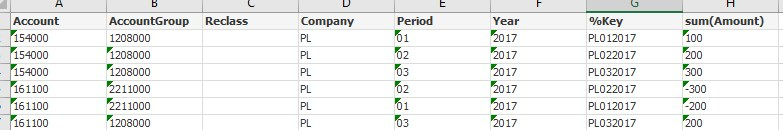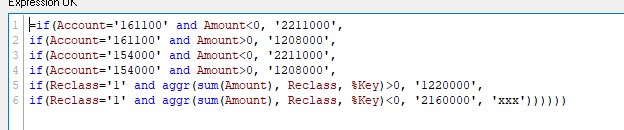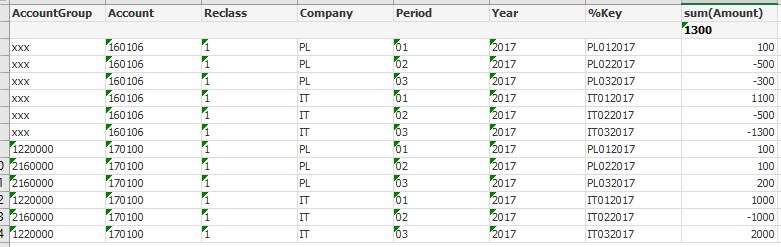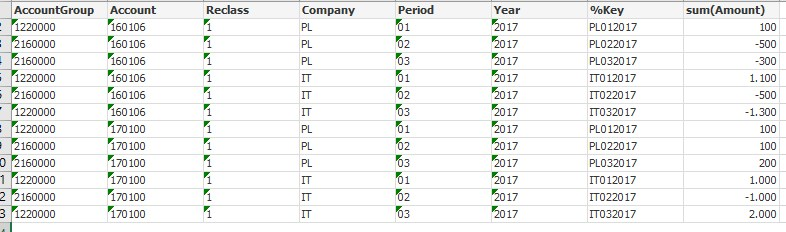# QlikView App Dev

Discussion Board for collaboration related to QlikView App Development.

Announcements
cancel
Showing results for
Did you mean:Contributor III

## If - Sum - Aggr Formula

Dear all,

we have been loading the accounts from the balance sheet into Qlikview and I want to show some accounts depending on the +/- side either on the active or liability side. The trigger is sometimes the positive or negative total of some accounts.

The first example is working fine - if the amount by company, Year, Period, Account is less than 0, then it should go to Accountgroup 2211000 if it is positive, then to 1208000:For the accounts 160106 and 170100, the total of both is giving the direction (reclass group 1) - if it is less than 0 to 2160000 and if it is positive than to 1220000.

With the formula I've applied:Qlikview uses the total of both to decide which account group to use, however this is only displayed in the line of one account (170100), but not on the level of 160106 - this one gets the 'xxx'.What do I need to change in the formula, so that the account 160106 gets as well the AccountGroup attribute?

Many thansk to you!

1 Solution

Accepted SolutionsMVP

Try this for your calculated dimension

=if(Account='161100' and Amount<0, '2211000',

if(Account='161100' and Amount>0, '1208000',

if(Account='154000' and Amount<0, '2211000',

if(Account='154000' and Amount>0, '1208000',

if(Reclass='1' and aggr(NODISTINCT sum(Amount), Reclass, %Key)>0, '1220000',

if(Reclass='1' and aggr(NODISTINCT sum(Amount), Reclass, %Key)<0, '2160000', 'xxx'))))))7 RepliesMVP

Not entirely sure I understand what you are after, would you be able to let us know what the expected output needs to look like?Contributor III
Author

Of course - I'm expecting this:MVP

Try this for your calculated dimension

=if(Account='161100' and Amount<0, '2211000',

if(Account='161100' and Amount>0, '1208000',

if(Account='154000' and Amount<0, '2211000',

if(Account='154000' and Amount>0, '1208000',

if(Reclass='1' and aggr(NODISTINCT sum(Amount), Reclass, %Key)>0, '1220000',

if(Reclass='1' and aggr(NODISTINCT sum(Amount), Reclass, %Key)<0, '2160000', 'xxx'))))))Contributor III
Author

It's working!

Many many thanks to you!

Would you mind explaining me what the Nodistinct is doing? I never heard about it....MVPContributor III
Author

Thank you!MVP

No problem at all